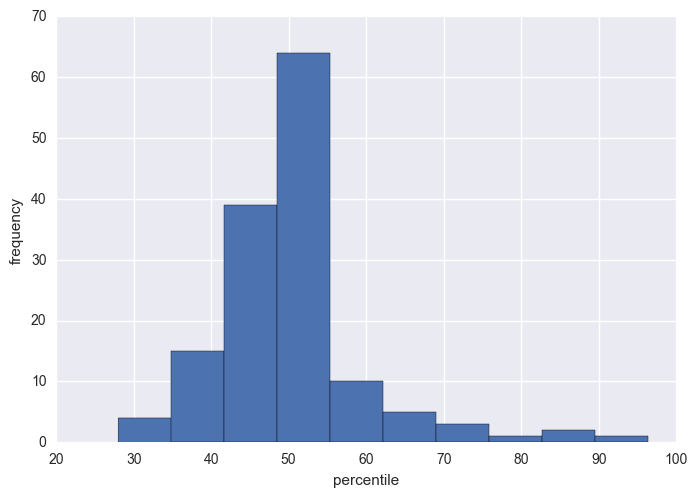## KQ3: Pritts Meta-analysis Update¶

In key question 3, we address the risk of sarcoma dissemination following morcellation of fibroids. We identified a recently published analysis conducted by Elizabeth Pritts and her colleagues (2015) to estimate the prevalence of occult leiomyosarcoma at time of treatment for presumed benign tumors (fibroids). We updated their search and used similar eligibility criteria to identify papers published since 2014. We extracted from these papers the number of women who were treated for uterine fibroids and the cases of leiomyosarcoma subsequently identified. We have combined these data with the data from the 134 publications that Pritts et al included in their analysis for a total of LMS rates from 148 sources.

In :
%matplotlib inline
import numpy as np
import pandas as pd
import pymc3 as pm
import seaborn as sns
import matplotlib.pyplot as plt
import pdb
sns.set()

rseeds = 20090425, 19771114

In :
kq3_data = pd.read_excel('data/Pritts_EPC_KQ3_Includes_Histopathology_SK_12142016.xlsx',
sheetname='Updated_Pritts_With_EPC_INclude',
na_values=['NR', 'NA'],
index_col=0)[['Author', 'Year', 'Design', 'Procedure', 'Indication',
'Age, Mean', 'Age, SD', 'Age, Median', 'Age, Min', 'Age, Max', 'Age, Other',
'LMS','Population','Tumors','InPritts','POSTOP_HP_Done']].dropna(thresh=7)

Out:
Author Year Design Procedure Indication Age, Mean Age, SD Age, Median Age, Min Age, Max Age, Other LMS Population Tumors InPritts POSTOP_HP_Done
Line
1.0 Adelusola KA, Ogunniyi SO 2001 Retrospective NE NE NaN NaN NaN 19.0 89 NaN 0 177 0 1.0 yes
2.0 Ahmed AA, Stachurski J, Abdel Aziz E et al 2002 Prospective NE NE NaN NaN NaN 29.0 65 NaN 0 10 0 1.0 yes
3.0 Angle HS, Cohen SM, Hidlebaugh D 1995 Retrospective NE NE 41.0 NaN NaN 24.0 81 NaN 0 41 0 1.0 yes
4.0 Banaczek Z, Sikora K, Lewandowska-Andruszuk I 2004 Retrospective NE NE 44.5 NaN NaN 29.0 73 NaN 0 309 0 1.0 yes
5.0 Barbieri R, Dilena M, Chumas J, Rein MS, Fried... 1993 RCT NE NE 33.7 NaN NaN NaN NaN 36.3 0 15 0 1.0 yes

Missing values

In :
kq3_data.isnull().sum()

Out:
Author              0
Year                0
Design              0
Procedure           0
Indication          1
Age, Mean          72
Age, SD           150
Age, Median       151
Age, Min           65
Age, Max           66
Age, Other        128
LMS                 0
Population          0
Tumors              0
InPritts            1
POSTOP_HP_Done      0
dtype: int64
In :
kq3_data['age_max'] = kq3_data['Age, Max'].replace('50+', 50)
kq3_data = kq3_data.rename(columns={'Age, Min': 'age_min',
'Age, Mean': 'age_mean',
'Age, SD': 'age_sd',
'Age, Median': 'age_med'})

In :
kq3_data.describe()

Out:
Year age_mean age_sd age_med age_min LMS Population Tumors InPritts age_max
count 162.000000 90.000000 12.000000 11.000000 97.00000 162.000000 162.000000 162.000000 161.000000 96.000000
mean 2005.185185 41.919222 7.009167 41.545455 26.91134 2.500000 975.851852 2.500000 0.832298 61.466667
std 7.858391 6.130203 2.821758 5.791954 6.20752 13.239903 3890.629500 13.239903 0.374767 13.430732
min 1984.000000 28.200000 1.800000 35.000000 18.00000 0.000000 5.000000 0.000000 0.000000 34.000000
25% 1999.000000 37.310000 5.925000 37.450000 22.00000 0.000000 40.250000 0.000000 1.000000 51.000000
50% 2006.000000 42.200000 6.600000 40.000000 26.00000 0.000000 92.000000 0.000000 1.000000 60.000000
75% 2012.000000 46.000000 7.697500 45.800000 31.00000 0.000000 359.500000 0.000000 1.000000 70.250000
max 2016.000000 59.600000 12.500000 52.900000 44.00000 115.000000 34728.000000 115.000000 1.000000 96.000000
In :
kq3_data[[c for c in kq3_data.columns if c.startswith('age')]].hist();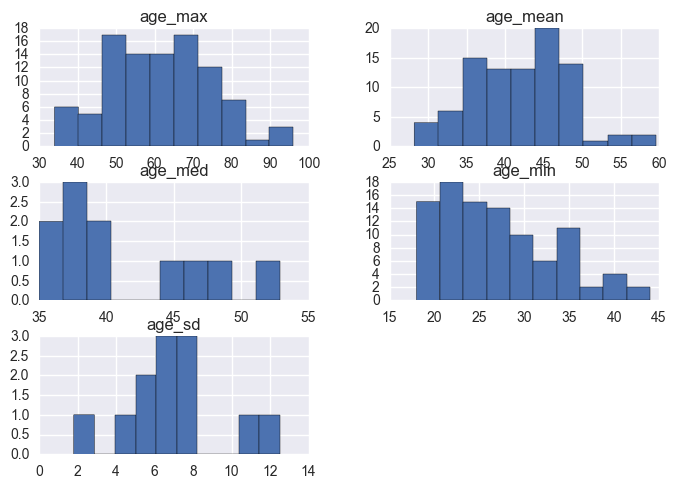Breakdown on studies by design.

In :
kq3_data['Design'] = kq3_data.Design.str.strip()

In :
kq3_data.Design.value_counts()

Out:
Retrospective    95
Prospective      39
RCT              27
prospective       1
Name: Design, dtype: int64

Combine prospective and RCT data into a single indicator.

In :
kq3_data['Prospective'] = (kq3_data.Design!='Retrospective').astype(int)


Following Pritts et al. (2015), I fit a binomial random effects model, such that event probabilities on the logit scale are normally distributed with mean $\mu$ and standard deviation $\sigma$. This distribution describes how the probabilities vary across studies, with the degree of variation described by $\sigma$.

$$\theta_i \sim N(\mu, \sigma^2)$$

the expected value for study $i$ is then inverse-logit transformed, and used as the event probability $\pi_i$ in a binomial model describing the number of observed tumors $t$:

$$\log\left[\frac{\pi_i}{1-\pi_i}\right] = \theta_i$$

$$t_i \sim \text{Bin}(n_i, \pi_i)$$

In :
import theano.tensor as tt
from numpy.ma import masked_values

biopsy_only = True

if biopsy_only:
model_data = kq3_data[kq3_data.POSTOP_HP_Done=='yes']
else:
model_data = kq3_data

k = model_data.shape
tumors = model_data.Tumors.values.astype(int)
n = model_data.Population.values.astype(int)
X = model_data['Prospective'].values.astype(int)
age_max_norm = ((model_data.age_max - 60)/10).fillna(0.5).values

poly_terms = 3

invlogit = pm.math.invlogit


There appears to be no obvious relationship between age (normalized) and tumor rate (though a quadratic model might be worth considering). We will not include age as a covariate in the model.

In :
plt.plot(age_max_norm, tumors/n, 'ro', alpha=0.3)

Out:
[<matplotlib.lines.Line2D at 0x11714da58>]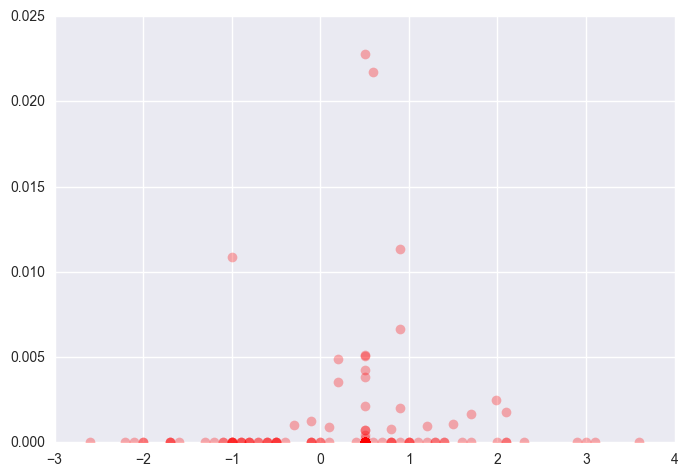In :
with pm.Model() as pritts_update:

# Study random effect
μ = pm.Normal('μ', 0, sd=100, testval=-3)
σ = pm.Uniform('σ', 0, 1000, testval=10)
θ = pm.Normal('θ', μ, sd=σ, shape=k)
# Design effects
β = pm.Normal('β', 0, sd=10)

# Study-specific probabilities
π = pm.Deterministic('π', invlogit(θ + β*X))

# Expected probabilities by design
p_retro_10000 = pm.Deterministic('p_retro_10000', invlogit(μ)*10000)
p_prosp_10000 = pm.Deterministic('p_prosp_10000', invlogit(μ + β)*10000)

obs = pm.Binomial('obs', n=n, p=π, observed=tumors)

In :
iterations = 5000
keep = 2000

In :
with pritts_update:
trace = pm.sample(iterations, random_seed=[20140425, 19700903])

Auto-assigning NUTS sampler...
Initializing NUTS using advi...
Average ELBO = -118.16: 100%|██████████| 200000/200000 [00:27<00:00, 7284.30it/s]
Finished [100%]: Average ELBO = -119.64
100%|██████████| 5000/5000 [00:17<00:00, 414.54it/s]


The following plots are the distribution of samples from the posterior distributions for the expected (population) probability of tumor (p_update), the inverse-logit expected probability ($\mu$) and the standard deviation of the probabilities on the inverse-logit scale ($\sigma$).

In :
pm.traceplot(trace[-keep:], varnames=['p_retro_10000', 'p_prosp_10000', 'μ', 'σ']);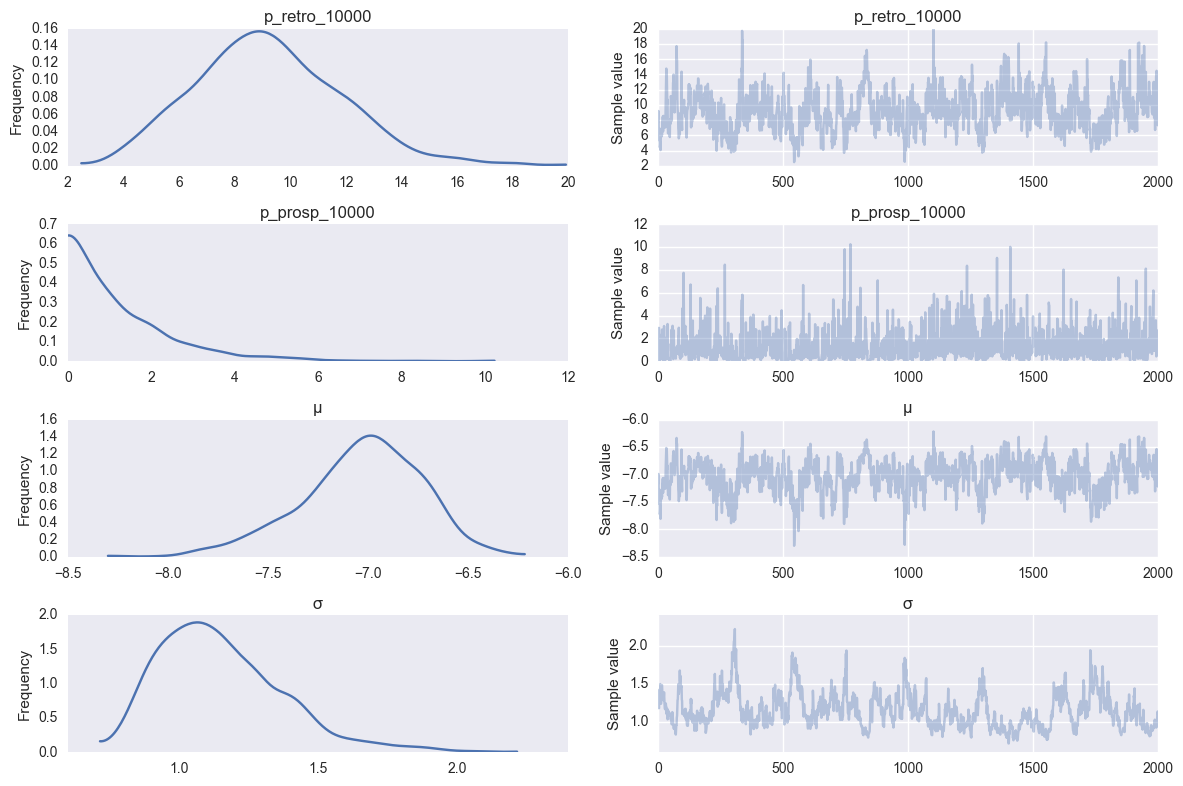Summary statistics from each of the above parameters.

In :
pm.summary(trace[-keep:], varnames=['p_retro_10000', 'p_prosp_10000', 'μ', 'σ'], roundto=4)

p_retro_10000:

Mean             SD               MC Error         95% HPD interval
-------------------------------------------------------------------

9.2213           2.6415           0.1869           [3.8420, 13.8150]

Posterior quantiles:
2.5            25             50             75             97.5
|--------------|==============|==============|--------------|

4.5703         7.4206         9.0588         10.9094        14.9693

p_prosp_10000:

Mean             SD               MC Error         95% HPD interval
-------------------------------------------------------------------

1.3419           1.3473           0.0405           [0.0020, 3.9332]

Posterior quantiles:
2.5            25             50             75             97.5
|--------------|==============|==============|--------------|

0.0364         0.3704         0.9296         1.8814         4.9647

μ:

Mean             SD               MC Error         95% HPD interval
-------------------------------------------------------------------

-7.0309          0.3003           0.0216           [-7.7169, -6.5332]

Posterior quantiles:
2.5            25             50             75             97.5
|--------------|==============|==============|--------------|

-7.6903        -7.2053        -7.0057        -6.8196        -6.5028

σ:

Mean             SD               MC Error         95% HPD interval
-------------------------------------------------------------------

1.1507           0.2241           0.0195           [0.7943, 1.6089]

Posterior quantiles:
2.5            25             50             75             97.5
|--------------|==============|==============|--------------|

0.8260         0.9857         1.1137         1.2762         1.7015



By comparison, estimates from Pritts' supplement:In :
p_vars = ['p_retro_10000', 'p_prosp_10000']
intervals = [pm.stats.hpd(trace[var][-keep:]) for var in p_vars]

In :
meds = [np.median(trace[var][-keep:]) for var in p_vars]
upper = [i - m for m,i in zip(meds, intervals)]
lower = [m - i for m,i in zip(meds, intervals)]

plt.errorbar(np.arange(2)-0.1, meds, yerr=[lower, upper], fmt='s', label='Updated MA')
plt.errorbar(np.arange(2)+0.1, [5.7, 1.2], yerr=[[5.7-1.7, 1.2], [11.3 - 5.7,7.5 - 1.2]],
color='r', fmt='D', label='Pritts MA', elinewidth=0.5)
plt.xticks(range(3), ['Retrospective', 'Prospective'])
plt.ylabel('Rate per 10,000 surgeries')
plt.xlim(-0.5, 1.5)
plt.legend();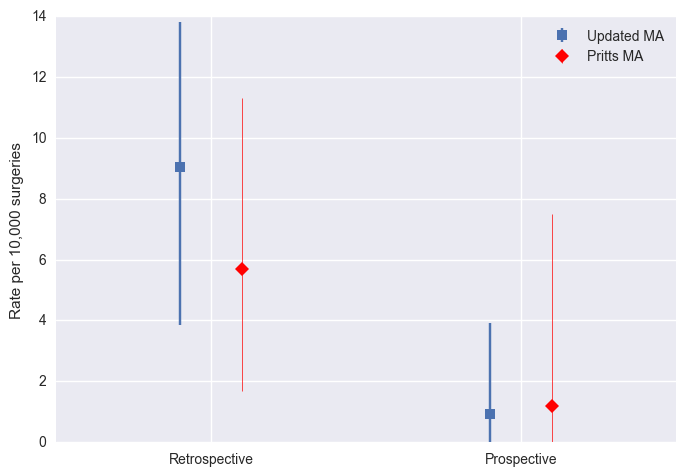### Goodness of fit¶

To check how well the model fits the data, I conducted posteior predictive checks. This simulates data from the model for each data point (i.e. study) in the meta-analysis, and compares the distribution of these simulated values (here, 500 replicates) with the value of the data itself. If the percentile of the datum is very extreme (either large or small), then it is evidence that the model does not adequately fit the data. The distribution of percentiles in the bottom histogram shows that there is no evidence of lack of fit.

In :
ppc = pm.sample_ppc(trace, model=pritts_update, samples=500)

100%|██████████| 500/500 [00:02<00:00, 180.22it/s]    | 12/500 [00:00<00:04, 118.72it/s]

In :
from scipy.stats import percentileofscore

p = [percentileofscore(s, o).round(2) for s,o in zip(ppc['obs'].T, tumors)]

plt.hist(p)
plt.xlabel('percentile')
plt.ylabel('frequency');